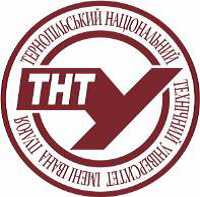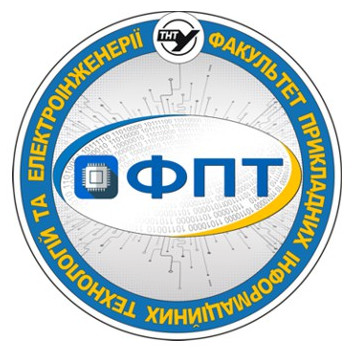## SyllabusTernopil Ivan Puluj National Technical UniversityКаф. електричної інженерії

Fundamentals of Electrical Engineering

syllabus

## 1. Educational programs for which discipline is mandatory:

# Educational stage Broad field Major Educational program Course(s) Semester(s)
1 bachelor's 14. Електрична інженерія 141. Електроенергетика, електротехніка та електромеханіка (бакалавр) 3 5

## 3. Information about the author of the course

Full name Zakordonets Volodymyr
Link to the teacher`s page on the official website of the University http://library.tntu.edu.ua/personaliji/a/z/zakordonec-volodymyr-savych/
Е-mail (in the domain tntu.edu.ua)

## 4. Information about the course

Study hours structure Lectures: 16
Practical classes: 16
Laboratory classes: 32

Amount of hours for individual work: 56
ECTS credits: 4
Teaching language english
Form of final examination exam
Link to an electronic course on the e-learning platform of the university https://dl.tntu.edu.ua/bounce.php?course=5395

## 5. Program of discipline

### The place of academic discipline in the structural and logical scheme of study according to the educational program

#### Prerequisites. List of disciplines, or knowledge and skills, possession of which students needed (training requirements) for successful discipline assimilation

Formation of general cultural and professional competences necessary for obtaining knowledge about electromagnetic phenomena and processes in electrical and electromagnetic devices and apparatuses

#### List of disciplines based on learning results from this discipline

Power Plants
Electrical Machines

### Contents of the academic discipline

#### Lectures (titles/topics)

Тheme 1. Basic laws and equations of the electrostatic field.
Spot charge. The Coulomb law. Voltage and potential of the electrostatic field. The principle of superposition. Condition of field potential in integral and differential form. Electric induction.
Тheme 2. Methods of calculating the electrostatic field.
Gauss's theorem for an electrostatic field in an integral and differential form. Equation of Poisson and Laplace. Boundary conditions on the boundary of two dielectrics. The energy of the electrostatic field.
Тheme 3. Basic laws and equations of the electric field of a direct current.
Current and current density. The law of Oma and Joule in a differential form. Kirchoff's first and second laws in a differential form. Boundary conditions on the separation surface of two conductors. Method of electrostatic analogy. Investigation of current emission in a conducting environment. Field of spherical electrode. Ground resistance.
Тheme 4. Basic laws and equations of the magnetic field of direct current.
Induction and magnetic field strength. The Bio-Savar-Laplace law. The law of full current in integral and differential forms. Magnetic flux. The principle of continuity of the magnetic flux in the integral and differential form. Scalar and vector magnetic potential.
Тheme 5. Methods of calculating the magnetic field.
Poisson equation for scalar and vector potentials. Magnetic voltage. Dependence between magnetic flux and vector potential. An analogy between electric and magnetic fields. Boundary conditions on the separation surface of two magnets. The method of mirror images. Magnetic field energy.
Тheme 6. Basic laws of the electromagnetic field.
Maxwell's first and second hypotheses. Conduction current, bias current, full current. Continuity equation of full current. The first and second Maxwell equations in integral and differential forms.
Тheme 7. Basic equations of electromagnetic field.
The third and fourth Maxwell equations in integral and differential forms. Complete system of Maxwell equations in dielectric and conducting environments. The energy of the electromagnetic field. The Poynting Theorem. Electrodynamic potentials of the electromagnetic field.
Тheme 8. Flat waves.
Distribution of plane waves. Wave equations. Symbolic method. Maxwell's equation in a symbolic form. Flat wave’s Flat wave equation. Distribution ratio. Fading factor and phase. Wavelength and phase velocity. Wave resistance.

#### Practical classes (topics)

Тheme 1. Basic laws and equations of the electrostatic field.
Spot charge. The Coulomb law. Voltage and potential of the electrostatic field. The principle of superposition. Condition of field potential in integral and differential form. Electric induction.
Тheme 2. Methods of calculating the electrostatic field.
Gauss's theorem for an electrostatic field in an integral and differential form. Equation of Poisson and Laplace. Boundary conditions on the boundary of two dielectrics. The energy of the electrostatic field.
Тheme 3. Basic laws and equations of the electric field of a direct current.
Current and current density. The law of Oma and Joule in a differential form. Kirchoff's first and second laws in a differential form. Boundary conditions on the separation surface of two conductors. Method of electrostatic analogy. Investigation of current emission in a conducting environment. Field of spherical electrode. Ground resistance.
Тheme 4. Basic laws and equations of the magnetic field of direct current.
Induction and magnetic field strength. The Bio-Savar-Laplace law. The law of full current in integral and differential forms. Magnetic flux. The principle of continuity of the magnetic flux in the integral and differential form. Scalar and vector magnetic potential.
Тheme 5. Methods of calculating the magnetic field.
Poisson equation for scalar and vector potentials. Magnetic voltage. Dependence between magnetic flux and vector potential. An analogy between electric and magnetic fields. Boundary conditions on the separation surface of two magnets. The method of mirror images. Magnetic field energy.
Тheme 6. Basic laws of the electromagnetic field.
Maxwell's first and second hypotheses. Conduction current, bias current, full current. Continuity equation of full current. The first and second Maxwell equations in integral and differential forms.
Тheme 7. Basic equations of electromagnetic field.
The third and fourth Maxwell equations in integral and differential forms. Complete system of Maxwell equations in dielectric and conducting environments. The energy of the electromagnetic field. The Poynting Theorem. Electrodynamic potentials of the electromagnetic field.
Тheme 8. Flat waves.
Distribution of plane waves. Wave equations. Symbolic method. Maxwell's equation in a symbolic form. Flat wave’s Flat wave equation. Distribution ratio. Fading factor and phase. Wavelength and phase velocity. Wave resistance.

#### Laboratory classes (topics)

Laboratory work 1.
Calculation of the field of a charged rod with the help of PC.
Laboratory work 2.
Simulation of the electric field by a quasi-stationary electric field of current in a conducting medium.
Laboratory work 3.
Investigation of current emission in a conducting environment.
Laboratory work 4.
Investigation of the magnetic field of the solenoid coil.
Laboratory work 5.
Investigation of a stationary magnetic field using Hall sensors.
Laboratory work 6.
Modeling of the permanent magnetic field of an electric machine by a stationary electric field in a conducting medium.
Laboratory work 7.
Investigation of the mutual inductance of round coils.
Laboratory work 8.
Investigation of conduction currents and displacement in a dielectric environment.
Approved by the department
(protocol №
on «
»
y.).